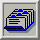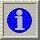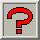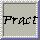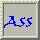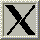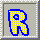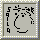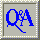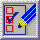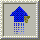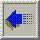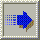##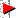3.9. Uniform Quantisation

From Glassner (1990) and Heckbert (1982) :

The simplest way to solve the problem of colour quantisation is using the method of Uniform Quantisation. This involves dividing the RGB cube into equal slices in each dimension and using the cross product of these (few) colour levels of every primary for the colour table. In other words, it is a bit like assuming you can adequately determine the population of each country in the world by dividing the world's total population by the number of countries !!

Although this is computationally much faster than other methods, it leaves much room for improvement, as the final result is often poor.